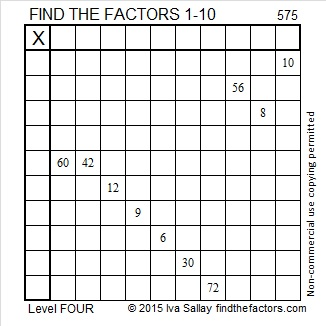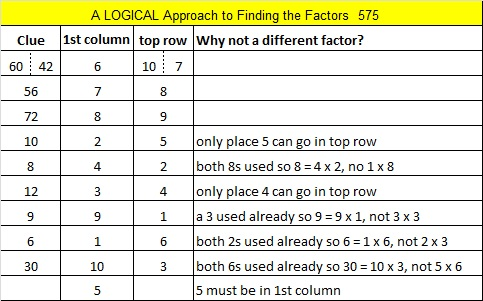# 575 and Level 4

575 is the hypotenuse of two Pythagorean triples: 345-460-575 and 161-552-575. What is the greatest common factor of each of those triples?

575 = 23 x 25, which can be written (24 – 1)(24 + 1) or (24^2) – 1.Print the puzzles or type the solution on this excel file: 10 Factors 2015-08-03

—————————————————————————————————

• 575 is a composite number.
• Prime factorization: 575 = 5 x 5 x 23, which can be written 575 = (5^2) x 23
• The exponents in the prime factorization are 2 and 1. Adding one to each and multiplying we get (2 + 1)(1 + 1) = 3 x 2  = 6. Therefore 575 has exactly 6 factors.
• Factors of 575: 1, 5, 23, 25, 115, 575
• Factor pairs: 575 = 1 x 575, 5 x 115, or 23 x 25
• Taking the factor pair with the largest square number factor, we get √575 = (√25)(√23) = 3√23 ≈ 23.9791576—————————————————————————————————This site uses Akismet to reduce spam. Learn how your comment data is processed.